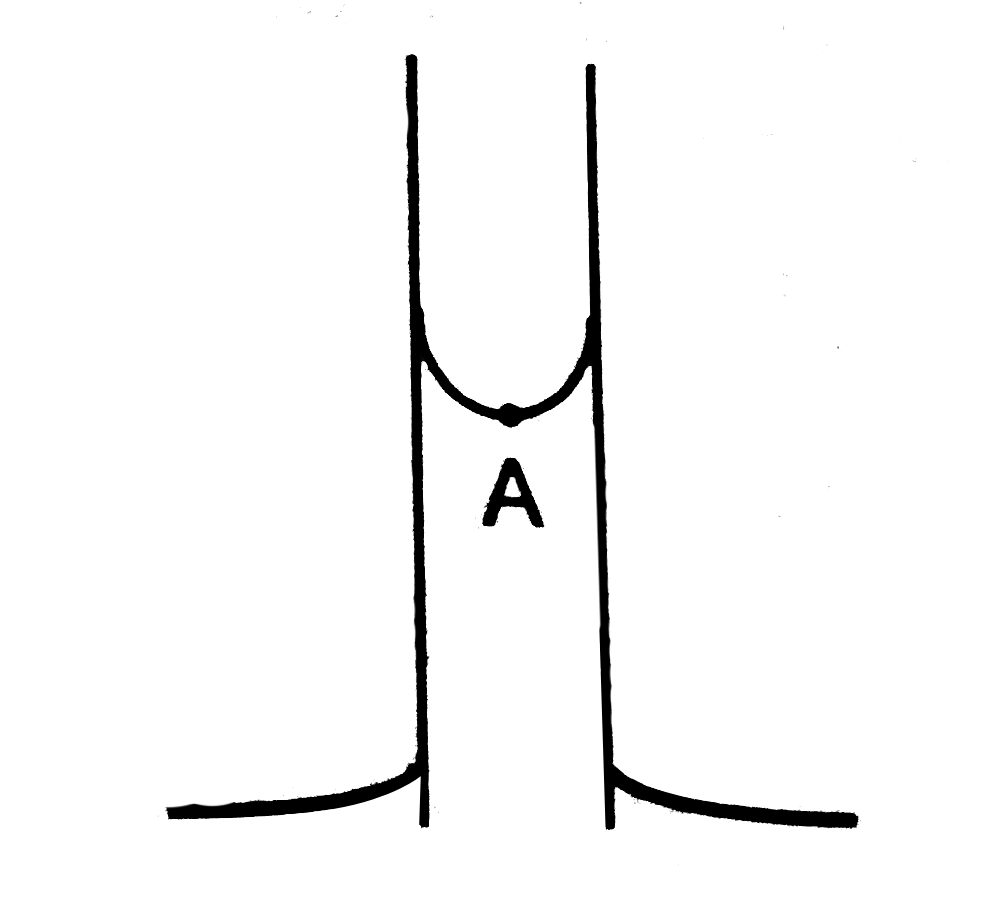# Figure shows a capillary tube of radius r dipped into water. If the atmosphere pressure is P_0, the pressure at point A is

35 views
in Physics
closed
Figure shows a capillary tube of radius r dipped into water. If the atmosphere pressure is P_0, the pressure at point A isA. P_(0)
B. P_(0)+(2s)/r
C. P_(0)-(2s)/r
D. P_(0)-(4s)/r

by (87.1k points)
selected

P_(A)=P_(0)-(2S)/r
The pressure inside a concacve meniscus is less than the pressure outside (atmosphere). Assuming the meiscus to be spherical (as for thin capillaries) excess pressure is 2S//r where r is the radius of the hemispherical surface.